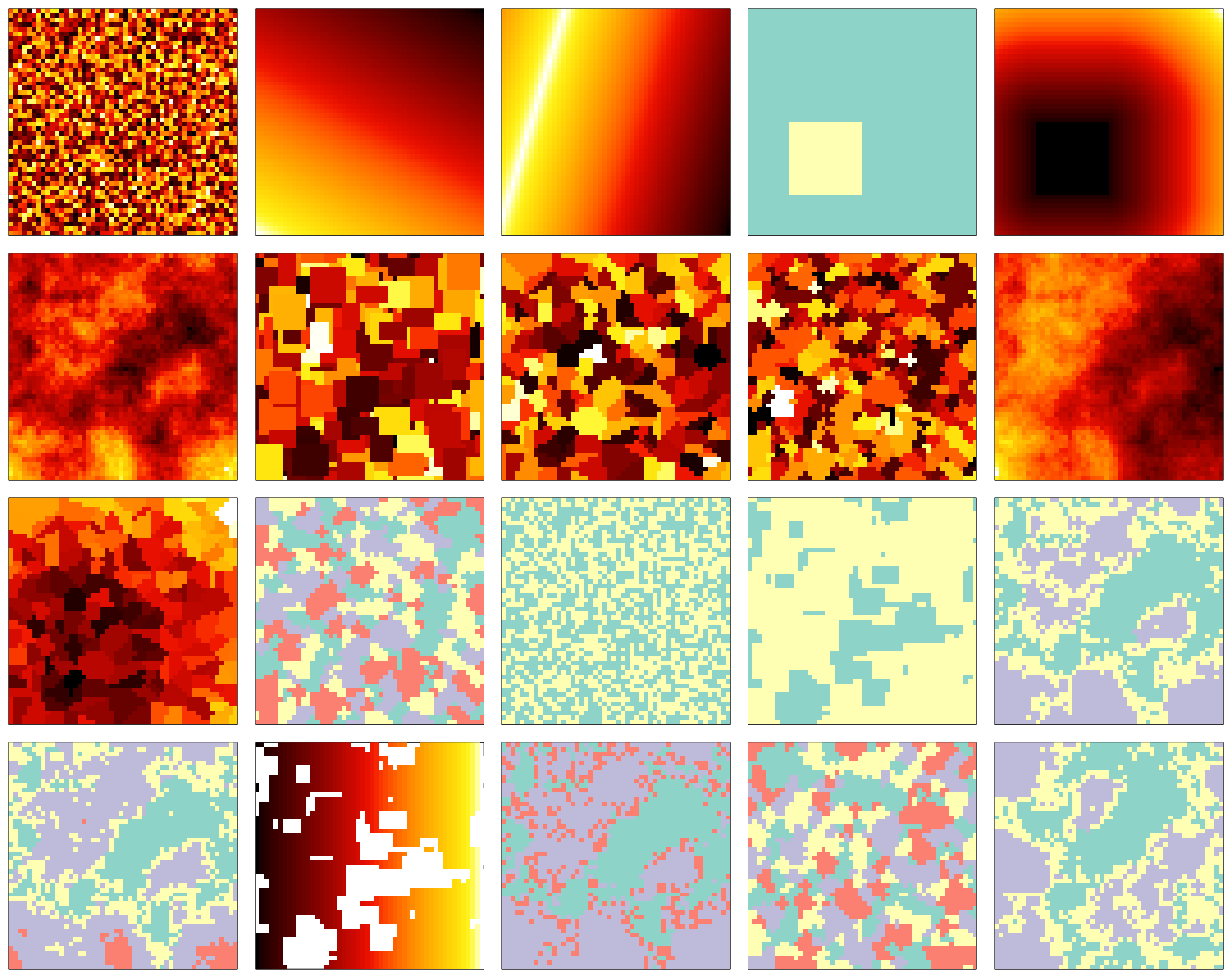## NeutralLandscapes.jl

Generation of neutral landscapes in Julia.
Author EcoJulia
Popularity
6 Stars
Updated Last
2 Years Ago
Started In
February 2021

# Neutral Landscapes

This packages allows the generation of neutral landscapes in Julia. It is a port of the `NLMPy` package, which is described in greater detail at:

Etherington, T.R., Holland, E.P. & O’Sullivan, D. (2015) NLMpy: a python software package for the creation of neutral landscape models within a general numerical framework. Methods in Ecology and Evolution, 6, 164–168.

All landscapes are generated using an overload of the `rand` (or `rand!`) method, taking as arguments a `NeutralLandscapeGenerator`, as well as a dimension and a Boolean mask if required. The additional functions `classify` and `blend` are used to respectively discretize the network, or merge the result of different neutral generators.

The code below reproduces figure 1 of Etherington et al. (2015):

```using NeutralLandscapes, Plots
siz = 50, 50

# Random NLM
Fig1d = falses(siz)
Fig1d[10:25, 10:25] .= true
# Midpoint displacement NLM
Fig1f = rand(MidpointDisplacement(0.75), siz)
# Random rectangular cluster NLM
Fig1g = rand(RectangularCluster(4, 8), siz)
# Random element nearest-neighbor NLM
Fig1h = rand(NearestNeighborElement(200), siz)
# Random cluster nearest-neighbor NLM
Fig1i = rand(NearestNeighborCluster(0.4), siz)
# Blended NLM
Fig1j = blend([Fig1f, Fig1c])
# Patch blended NLM
Fig1k = blend(Fig1h, Fig1e, 1.5)
# Classifiend random cluster nearest-neighbor NLM
Fig1l = classify(Fig1i, ones(4))
# Percolation NLM
Fig1m = classify(Fig1a, [1-0.5, 0.5])
# Binary random rectangular cluster NLM
Fig1n = classify(Fig1g, [1-0.75, 0.75])
# Classified midpoint displacement NLM
Fig1o = classify(Fig1f, ones(3))
# Classified midpoind displacement NLM, with limited classification
Fig1p = classify(Fig1f, ones(3), Fig1d)
Fig1q = rand(PlanarGradient(90), siz, mask = Fig1n .== 2) #TODO mask as keyword + should mask be matrix or vec or both? (Fig1e)
# Hierarchical NLM
Fig1r = ifelse.(Fig1o .== 2, Fig1m .+ 2, Fig1o)
# Rotated NLM
Fig1s = rotr90(Fig1l)
# Transposed NLM
Fig1t = Fig1o'

class = cgrad(:Set3_4, 4, categorical = true)
c2, c3, c4 = class[1:2], class[1:3], class[1:4]

gr(color = :fire, ticks = false, framestyle = :box, colorbar = false)
plot(
heatmap(Fig1a),         heatmap(Fig1b),         heatmap(Fig1c),         heatmap(Fig1d, c = c2), heatmap(Fig1e),
heatmap(Fig1f),         heatmap(Fig1g),         heatmap(Fig1h),         heatmap(Fig1i),         heatmap(Fig1j),
heatmap(Fig1k),         heatmap(Fig1l, c = c4), heatmap(Fig1m, c = c2), heatmap(Fig1n, c = c2), heatmap(Fig1o, c = c3),
heatmap(Fig1p, c = c4), heatmap(Fig1q),         heatmap(Fig1r, c = c4), heatmap(Fig1s, c = c4), heatmap(Fig1t, c = c3),
layout = (4,5), size = (1600, 1270)
)```### Used By Packages

No packages found.